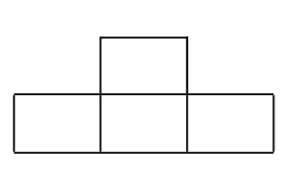MTH4150 Home

# MTH 4150: Midterm 1 Practice 1

Problem 1

In how many different ways we can assign offices to two employees if the total number of offices in the company is $$100$$?

Problem 2

How many different bit strings of length $$20$$ are there?

Problem 3

How many one-to-one functions are there from a set with $$m$$ elements to one with $$n$$ elements?

Problem 4

Determine the number of positive integers whose decimal expansion contains exactly 4 digits, but does not contain equal digits, and does not contain the digit $$3$$.

Problem 5

How many bit strings of length eight start with $$1$$ or end with the two bits $$00$$?

Problem 6

How many integers between $$1$$ and $$1000$$ have all their digits different and do not contain the digit $$5$$ in their decimal expansion?

Problem 7

Let $$S$$ be a subset of the set $$\{1,2,3,\dots, 2n\}$$. Assume that the equation $$x+y=2n+1$$ does not have solutions in $$S$$ (i.e., if $$x,y\in S$$, then $$x+y\neq 2n+1$$). What is the largest number of elements that $$S$$ can have?

Problem 8

Let $$n\geq 1$$ be a positive integer. If $$a_1, \dots, a_n$$ are positive integers, prove that it is possible to paint some of these numbers in green in such a way that the sum of green numbers is divisible by $$n$$.

Problem 9

In how many ways can we distribute 15 identical apples to 4 distinct students? Not all students have to get an apple.

Problem 10

Determine the total number of triples of nonnegative integers $$(a,b,c)$$ that satisfy $a+b+c=30.$

Problem 11

In how many different ways can we give 5 identical apples to 20 different students so that no student gets all 5 apples? (All apples are supposed to be given to students).

Problem 12

In how many different ways can we distribute $$15$$ identical apples to $$4$$ different students if not all of the apples have to be distributed?

Problem 13

Determine the number of subsets of $$\{1, 2, 3, 4, \dots, 50\}$$ whose sum of elements is larger than or equal to 638.

Problem 14

Let $$n > 3$$ be an integer. $$n$$ teams participated in a basketball tournament. Each team played one game with every other team. There were no ties. Prove that there exists a team $$A$$ such that: For every team $$B$$ that won a game against $$A$$, there exists a team $$C$$ such that $$A$$ won the game against $$C$$, and $$C$$ won the game against $$B$$.

Problem 15

Each of three schools contain $$n$$ students. Each student has at least $$n+1$$ friends among students of the other two schools. Prove that there are three students, all from different schools who are friends to each other. (Friendship is symmetric: If $$X$$ is a friend to $$Y$$, then $$Y$$ is a friend to $$X$$.)

Problem 16

A corner square is removed from the $$8\times 8$$ chessboard. Can the rest be tiled by $$1\times 3$$ rectangles?

Problem 17

• (a) Is it possible to tile the $$8\times 8$$ board using the figures congruent to?

• (b) Is it possible to tile the $$10\times 10$$ board using the figures congruent to?